Quick Contact# Forces and dynamics

### Forces and Dynamics

2.2.1 Calculate the weight of a body using the expression W = mg.

In general terms one can describe any force as 'the cause of a deformation or a velocity change'. A force is a vector quantity and the SI units for the measurement of force are Newton’s (N).

Weight
The weight of a body is the gravitational force experienced by that body. On earth the formula give is:

W = mg

- The mass of the body measured in kilograms (kg)

g - the gravitational field strength of the earth which is measured in Newton’s per kilogram (N kg-1) or sometimes m s-2. On the surface of the earth g= 9.81 N kg -1.

## 2.2.2 Identify the forces acting on an object and draw free-body diagrams representing the forces acting.

Tension
A string that is taut is said to be under tension. Therefore we can say tension (T) is the force that arises in any body when it is stretched. (Note that a string or rope that is not taut has zero tension in it).Figure 2.2.1 - Tension forces

Normal Reaction force:

If a body touches another body, there is a reaction force(R) between the two bodies. This force is perpendicular to the body exerting the force:Figure 2.2.2 - Normal reaction forces

Drag Force:

Drag forces are forces that oppose the motion of a body through a fluid (gas or liquid). They are directed opposite to the velocity of the body and generally depend on the speed of that body. Higher speed equals higher drag force.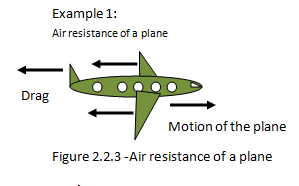Figure 2.2.3 -Air resistance of a plane

Up thrust:
An object placed in a fluid medium will experience up thrust. If the up thrust force on a body is equal to the weight, the body will float in the fluid.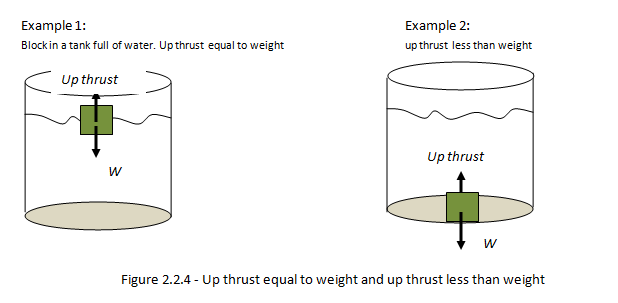Figure 2.2.4 - Up thrust equal to weight and up thrust less than weight

Frictional Forces:
Frictional forces (f) are forces that oppose the motion of a body f.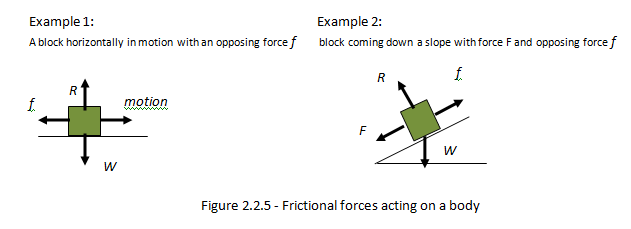Figure 2.2.5 - Frictional forces acting on a body

Hooke’s Law:

Hooke’s law states that up to the elastic limit, the extensionof a spring is proportional to thetension force, F. The constant of proportionality k is called the spring constant. SI units of spring constant are N m-1.

Mathematically,

F∞x

F = Kx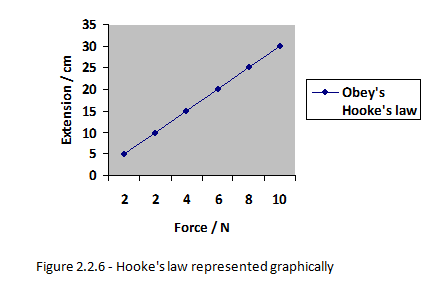Figure 2.2.6 - Hooke's law represented graphically

## 2.2.3 Determine the resultant force in different situations.

The resultant force is the overall force acting on an object when all the individual forces acting on that object have been added together.

In order to determine the resultant force acting upon an object, we need to add the individual forces. Consider figure 2.2.7 below: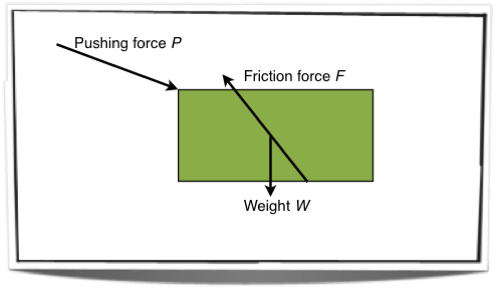Figure 2.2.7 - Forces acting on an object

Figure 2.2.7 shows the free body diagram of an object with three forces acting upon it. In order to determine the resultant force we must add these forces together. This is shown in figure 2.2.8 below: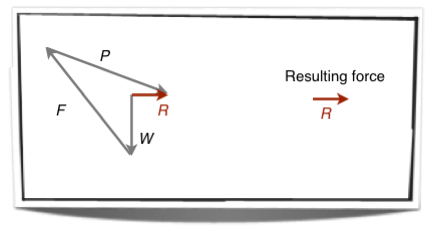Figure 2.2.8 - The resultant force

Figure 2.2.8 shows the resultant force attained through simple vector addition, for more on vector addition see section 1.3.2.

Content